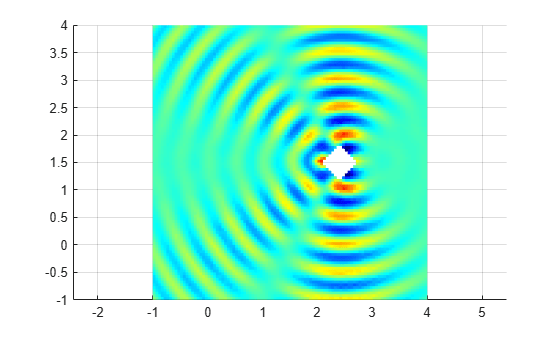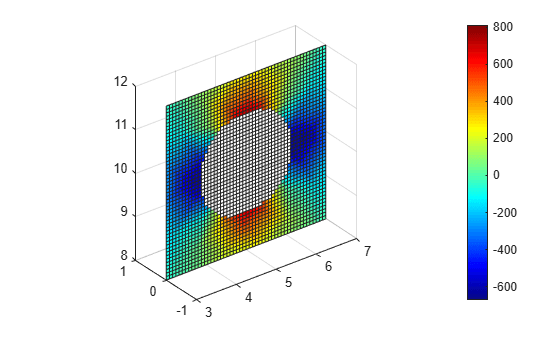# interpolateHarmonicField

Interpolate electric or magnetic field in harmonic result at arbitrary spatial locations

## Syntax

``EHintrp = interpolateHarmonicField(harmonicresults,xq,yq)``
``EHintrp = interpolateHarmonicField(harmonicresults,xq,yq,zq)``
``EHintrp = interpolateHarmonicField(harmonicresults,querypoints)``

## Description

example

````EHintrp = interpolateHarmonicField(harmonicresults,xq,yq)` returns the interpolated electric or magnetic field values at the 2-D points specified in `xq` and `yq`.```

example

````EHintrp = interpolateHarmonicField(harmonicresults,xq,yq,zq)` uses 3-D points specified in `xq`, `yq`, and `zq`.```

example

````EHintrp = interpolateHarmonicField(harmonicresults,querypoints)` returns the interpolated electric or magnetic field values at the points specified in `querypoints`.```

## Examples

collapse all

Solve a simple scattering problem and interpolate the x-component of the resulting electric field. A scattering problem computes the waves reflected by a square object illuminated by incident waves.

Create an electromagnetic model for harmonic analysis.

`emagmodel = createpde("electromagnetic","harmonic");`

Specify the wave number $\mathit{k}=\omega /\alpha$ as $4\pi$.

`k = 4*pi;`

Represent the square surface with a diamond-shaped hole. Define a diamond in a square, place them in one matrix, and create a set formula that subtracts the diamond from the square.

```square = [3; 4; -5; -5; 5; 5; -5; 5; 5; -5]; diamond = [2; 4; 2.1; 2.4; 2.7; 2.4; 1.5; 1.8; 1.5; 1.2]; gd = [square,diamond]; ns = char('square','diamond')'; sf = 'square - diamond';```

Create the geometry.

```g = decsg(gd,sf,ns); geometryFromEdges(emagmodel,g);```

Include the geometry in the model and plot it with the edge labels.

```figure; pdegplot(emagmodel,"EdgeLabels","on"); xlim([-6,6]) ylim([-6,6])```Specify the vacuum permittivity and permeability values as 1.

```emagmodel.VacuumPermittivity = 1; emagmodel.VacuumPermeability = 1;```

Specify the relative permittivity, relative permeability, and conductivity of the material.

```electromagneticProperties(emagmodel,"RelativePermittivity",1, ... "RelativePermeability",1, ... "Conductivity",0);```

Apply the absorbing boundary condition on the edges of the square. Specify the thickness and attenuation rate for the absorbing region by using the `Thickness`, `Exponent`, and `Scaling` arguments.

```electromagneticBC(emagmodel,"Edge",[1 2 7 8], ... "FarField","absorbing", ... "Thickness",2, ... "Exponent",4, ... "Scaling",1);```

Apply the boundary condition on the edges of the diamond.

```innerBCFunc = @(location,~) [-exp(-1i*k*location.x); zeros(1,length(location.x))]; bInner = electromagneticBC(emagmodel,"Edge",[3 4 5 6], ... "ElectricField",innerBCFunc);```

Generate a mesh.

`generateMesh(emagmodel,"Hmax",0.1);`

Solve the harmonic analysis model for the frequency `k` = $4\pi$.

`result = solve(emagmodel,"Frequency",k);`

Plot the real part of the x-component of the resulting electric field.

```u = result.ElectricField; figure pdeplot(emagmodel,"XYData",real(u.Ex),"Mesh","off"); colormap(jet)```Interpolate the resulting electric field to a grid covering the portion of the geometry, for x and y from -1 to 4.

```v = linspace(-1,4,101); [X,Y] = meshgrid(v); Eintrp = interpolateHarmonicField(result,X,Y);```

Reshape `Eintrp.Ex` and plot the x-component of the resulting electric field.

```EintrpX = reshape(Eintrp.ElectricField.Ex,size(X)); figure surf(X,Y,real(EintrpX),"LineStyle","none"); view(0,90) colormap(jet)```Interpolate the x-component of the magnetic field in a harmonic analysis of a 3-D model.

Create an electromagnetic model for harmonic analysis.

`emagmodel = createpde("electromagnetic","harmonic");`

By default, the field type is electric. Use the `FieldType` property of the model to change the field type to magnetic.

`emagmodel.FieldType = "magnetic";`

Import and plot the geometry representing a plate with a hole.

```importGeometry(emagmodel,"PlateHoleSolid.stl"); pdegplot(emagmodel,"FaceLabels","on","FaceAlpha",0.3)```Specify the vacuum permittivity and permeability values in the SI system of units.

```emagmodel.VacuumPermittivity = 8.8541878128E-12; emagmodel.VacuumPermeability = 1.2566370614E-6;```

Specify the relative permittivity, relative permeability, and conductivity of the material.

```electromagneticProperties(emagmodel,"RelativePermittivity",1, ... "RelativePermeability",6, ... "Conductivity",60);```

Specify the current density for the entire geometry. For harmonic analysis with the magnetic field type, the toolbox uses the curl of the specified current density.

`electromagneticSource(emagmodel,"CurrentDensity",[1;1;1]);`

Apply the absorbing boundary condition with a thickness of 0.1 on the side faces.

```electromagneticBC(emagmodel,"Face",3:6, ... "FarField","absorbing", ... "Thickness",0.1);```

Specify the magnetic field on the face bordering the round hole in the center of the geometry.

`electromagneticBC(emagmodel,"Face",7,"MagneticField",[1000;0;0]);`

Generate a mesh.

`generateMesh(emagmodel);`

Solve the model for a frequency of 50.

`result = solve(emagmodel,"Frequency",50);`

Plot the real part of the x-component of the resulting magnetic field.

```u = result.MagneticField; figure pdeplot3D(emagmodel,"ColorMapData",real(u.Hx)); colormap jet xlabel 'x' ylabel 'y' title("Real Part of x-Component of Magnetic Field")```Interpolate the resulting magnetic field to a grid covering the central portion of the geometry, for x, y, and z.

```x = linspace(3,7,51); y = linspace(0,1,51); z = linspace(8,12,51); [X,Y,Z] = meshgrid(x,y,z); Hintrp = interpolateHarmonicField(result,X,Y,Z)```
```Hintrp = struct with fields: MagneticField: [1x1 FEStruct] ```

Reshape H`intrp.Hx` and plot the x-component of the resulting magnetic field as a slice plot for y = 0.

```HintrpX = reshape(Hintrp.MagneticField.Hx,size(X)); figure slice(X,Y,Z,real(HintrpX),[],0,[],'cubic') axis equal colorbar colormap(jet)```Alternatively, you can specify the grid by using a matrix of query points.

```querypoints = [X(:),Y(:),Z(:)]'; Hintrp = interpolateHarmonicField(result,querypoints)```
```Hintrp = struct with fields: MagneticField: [1x1 FEStruct] ```

## Input Arguments

collapse all

Solution of a harmonic electromagnetic problem, specified as a `HarmonicResults` object. Create `harmonicresults` using the `solve` function.

Example: ```harmonicresults = solve(emagmodel,"Frequency",omega)```

x-coordinate query points, specified as a real array. `interpolateHarmonicField` evaluates the electric or magnetic field at the 2-D coordinate points `[xq(i) yq(i)]` or at the 3-D coordinate points `[xq(i) yq(i) zq(i)]` for every index `i`.Therefore, `xq`, `yq`, and (if present) `zq` must have the same number of entries.

`interpolateHarmonicField` converts the query points to column vectors `xq(:)`, `yq(:)`, and (if present) `zq(:)`. It returns electric or magnetic field values as a column vector of the same size. To ensure that the dimensions of the returned solution are consistent with the dimensions of the original query points, use `reshape`. For example, use ```EHintrpX = reshape(EHintrp.Ex,size(xq))```.

Example: `xq = [0.5 0.5 0.75 0.75]`

Data Types: `double`

y-coordinate query points, specified as a real array. `interpolateHarmonicField` evaluates the electric or magnetic field at the 2-D coordinate points `[xq(i) yq(i)]` or at the 3-D coordinate points `[xq(i) yq(i) zq(i)]` for every index `i`. Therefore, `xq`, `yq`, and (if present) `zq` must have the same number of entries.

`interpolateHarmonicField` converts the query points to column vectors `xq(:)`, `yq(:)`, and (if present) `zq(:)`. It returns electric or magnetic field values as a column vector of the same size. To ensure that the dimensions of the returned solution are consistent with the dimensions of the original query points, use `reshape`. For example, use ```EHintrpY = reshape(EHintrp.Ey,size(yq))```.

Example: `yq = [1 2 0 0.5]`

Data Types: `double`

z-coordinate query points, specified as a real array. `interpolateHarmonicField` evaluates the electric or magnetic field at the 3-D coordinate points `[xq(i) yq(i) zq(i)]` for every index `i`. Therefore, `xq`, `yq`, and `zq` must have the same number of entries.

`interpolateHarmonicField` converts the query points to column vectors `xq(:)`, `yq(:)`, and `zq(:)`. It returns electric or magnetic field values as a column vector of the same size. To ensure that the dimensions of the returned solution are consistent with the dimensions of the original query points, use `reshape`. For example, use ```EHintrpZ = reshape(EHintrp.Ez,size(zq))```.

Example: `zq = [1 1 0 1.5]`

Data Types: `double`

Query points, specified as a real matrix with either two rows for a 2-D geometry or three rows for a 3-D geometry. `interpolateHarmonicField` evaluates the electric or magnetic field at the coordinate points `querypoints(:,i)` for every index `i`, so each column of `querypoints` contains exactly one 2-D or 3-D query point.

Example: For a 2-D geometry, ```querypoints = [0.5 0.5 0.75 0.75; 1 2 0 0.5]```

Data Types: `double`

## Output Arguments

collapse all

Electric or magnetic field at query points, returned as an `FEStruct` object with the properties representing the spatial components of the electric or magnetic field at the query points. For query points that are outside the geometry, `EHintrp.Ex(i)`, `EHintrp.Ey(i)`, `EHintrp.Ez(i)`, `EHintrp.Hx(i)`, `EHintrp.Hy(i)`, and `EHintrp.Hz(i)` are `NaN`. Properties of an `FEStruct` object are read-only.

## Version History

Introduced in R2022a